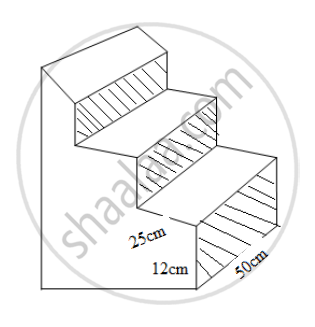There are three stair-steps as shown in the figure below. Each stair step has width 25 cm, height 12 cm and length 50 cm. How many bricks have been used in it, if each brick is 12.5 cm x 6.25 cm x 4 cm? - Geometry

There are three stair-steps as shown in the figure below. Each stair step has width 25 cm, height 12 cm and length 50 cm. How many bricks have been used in it, if each brick is 12.5 cm x 6.25 cm x 4 cm?Solution

The 1 st stair-step=h1=12 cm.

The 2 nd stair-step=h2=24 cm.

The 3 rd stair-step=h3=36 cm.

The total height=h= h1+ h2+ h3= 12 + 24 + 36 = 72 cm.

Length and width will remain same.

Length=l=50 cm

Width=w=25 cm

The volume of the three stair-step=Length(l) × Width(w) × Height(h)

The volume of the three stair-step=50 × 25 × 72 cu.cm

Given: The volume of 1 brick = 12.5 × 6.25 × 4 cu.cm

Let the number of bricks required be n.

(12.5 × 6.25 × 4) × n = 50 × 25× 72

n=(50xx25xx72)/(12.5xx6.25xx4)=288

288 bricks are used in three stair-steps.

Concept: Heights and Distances
Is there an error in this question or solution?